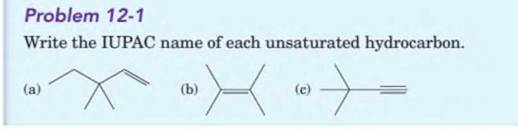# (a) Interpretation: The IUPAC name for unsaturated hydrocarbons should be determined. Concept Introduction: IUPAC rules are: Firstly, identify the longest chain of carbon atoms and give the name of the chain which is considered as the base name of the compound. Numbering of carbon atoms is done in such a way that the functional group and the substituent get the lowest number.BuyFind

### Introduction to General, Organic a...

11th Edition
Frederick A. Bettelheim + 4 others
Publisher: Cengage Learning
ISBN: 9781285869759BuyFind

### Introduction to General, Organic a...

11th Edition
Frederick A. Bettelheim + 4 others
Publisher: Cengage Learning
ISBN: 9781285869759

#### Solutions

Chapter 12.3, Problem 12.1P
Textbook Problem

##Expert Solution
Interpretation Introduction

(a)

Interpretation:

The IUPAC name for unsaturated hydrocarbons should be determined.

Concept Introduction:

IUPAC rules are:

1. Firstly, identify the longest chain of carbon atoms and give the name of the chain which is considered as the base name of the compound.
2. Numbering of carbon atoms is done in such a way that the functional group and the substituent get the lowest number.

### Explanation of Solution

First long carbon chain is labeled in numbers where functional carbon atoms get lowest number. Here, functional group is alkene group (double bond is the functional group).

Expert Solution
Interpretation Introduction

(b)

Interpretation:

The IUPAC name for unsaturated hydrocarbons should be determined.

Concept Introduction:

IUPAC rules are:

1. Firstly, identify the longest chain of carbon atoms and give the name of the chain which is considered as the base name of the compound.
2. Numbering of carbon atoms is done in such a way that the functional group and the substituent get the lowest number.
Expert Solution

(c)

Interpretation Introduction

Interpretation:

The IUPAC name for unsaturated hydrocarbons should be determined.

Concept Introduction:

IUPAC rules are:

1. Firstly, identify the longest chain of carbon atoms and give the name of the chain which is considered as the base name of the compound.
2. Numbering of carbon atoms is done in such a way that the functional group and the substituent get the lowest number.

## Expert Solution

### Want to see the full answer?

Check out a sample textbook solution.

### Want to see this answer and more?

Experts are waiting 24/7 to provide step-by-step solutions in as fast as 30 minutes!*

*Response times may vary by subject and question complexity. Median response time is 34 minutes for paid subscribers and may be longer for promotional offers.© 2021 bartleby. All Rights Reserved.